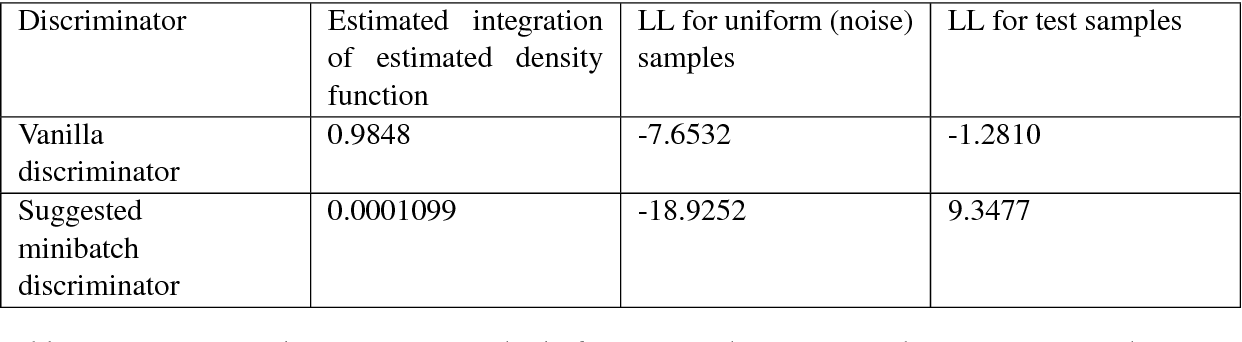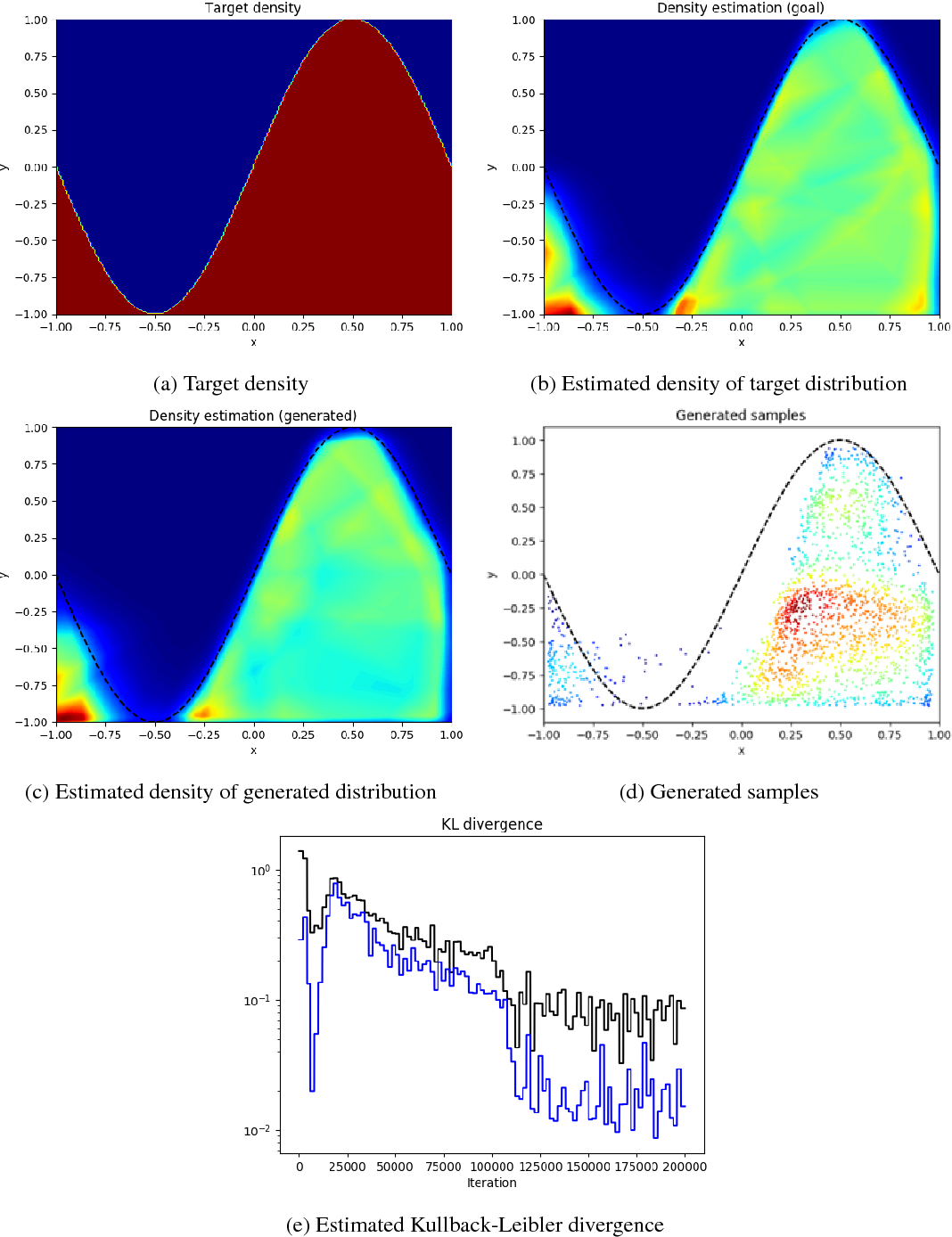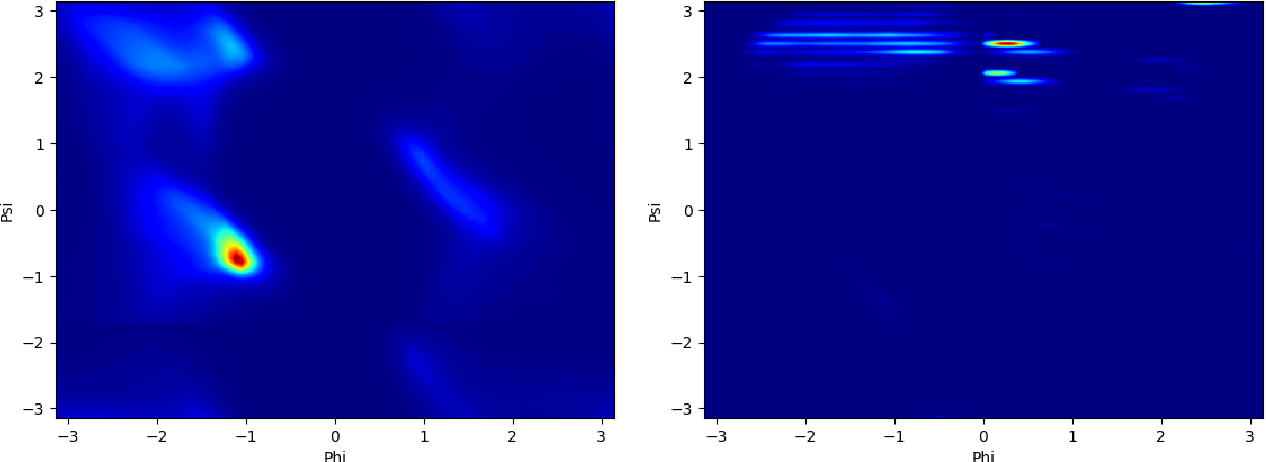Several dihedral angles prediction methods were developed for protein structure prediction and their other applications. However, distribution of predicted angles would not be similar to that of real angles. To address this we employed generative adversarial networks (GAN). Generative adversarial networks are composed of two adversarially trained networks: a discriminator and a generator. A discriminator distinguishes samples from a dataset and generated samples while a generator generates realistic samples. Although the discriminator of GANs is trained to estimate density, GAN model is intractable. On the other hand, noise-contrastive estimation (NCE) was introduced to estimate a normalization constant of an unnormalized statistical model and thus the density function. In this thesis, we introduce noise-contrastive estimation generative adversarial networks (NCE-GAN) which enables explicit density estimation of a GAN model. And a new loss for the generator is proposed. We also propose residue-wise variants of auxiliary classifier GAN (AC-GAN) and Semi-supervised GAN to handle sequence information in a window. In our experiment, the conditional generative adversarial network (C-GAN), AC-GAN and Semi-supervised GAN were compared. And experiments done with improved conditions were invested. We identified a phenomenon of AC-GAN that distribution of its predicted angles is composed of unusual clusters. The distribution of the predicted angles of Semi-supervised GAN was most similar to the Ramachandran plot. We found that adding the output of the NCE as an additional input of the discriminator is helpful to stabilize the training of the GANs and to capture the detailed structures. Adding regression loss and using predicted angles by regression loss only model could improve the conditional generation performance of the C-GAN and AC-GAN.

* 72 pages, MSc thesis under the supervision of Assoc. Prof. Thomas Hamelryck and Asst. Prof. Wouter BoomsmaClick To Get Code For Paper